# Correlation function in statistical mechanics

(diff) ← Older revision | Latest revision (diff) | Newer revision → (diff)

A function describing the influence of particles or groups of particles on one another and the effects due to the interaction of subsystems of the system under consideration.

In classical statistical mechanics, the correlation functions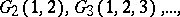are defined by the relations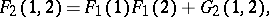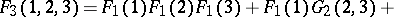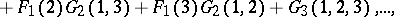where the symbols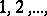in the arguments of the functions denote the sets of coordinatesand momenta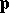of the 1st, 2ndparticles, respectively, and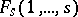are the reduced distribution functions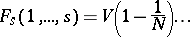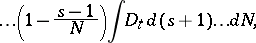whereis the volume of the system,is the number of particles and the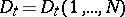are the distribution functions in the phase space at time, normalized so that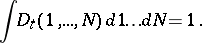The variation of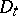in time is characterized by the Liouville equation, whererepresents the Liouville operator, which is not explicitly dependent on time. One usually considers the case in whichis the sum of an additive part and a binary part characterizing the interactions of the particles: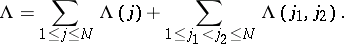According to the principle of correlation damping, the correlation functions satisfy the boundary conditions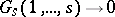as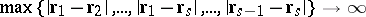.

The correlation functions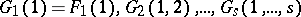are the functional derivatives,of a functional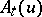which is related to the so-called generating functional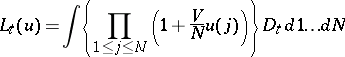by the relationThe functionalsatisfies the equation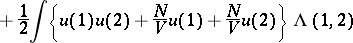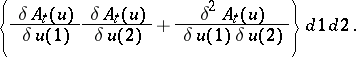In quantum statistical mechanics, the correlation functions are operator quantities, defined as follows:(*)where,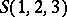are the symmetrization operator for Bose systems and the anti-symmetrization operator for Fermi systems. The correlation functions (*), forming the density matrix, satisfy the quantum-mechanical Liouville equation (see ).

In quantum statistical mechanics, besides the correlation function (*) one considers correlation functions based on conventional thermodynamical averages (see ), and correlation functions based on quasi-averages (see ).

Bilinear combinations of correlation functions (both quantum-mechanical and classical) yield the Green functions (see ). Correlation functions possess spectral representations; they satisfy the Bogolyubov inequality and a variation of the mean-value theorem (see ).

Correlation functions corresponding to the Kirkwood decomposition are sometimes used (see ); another version is a space-time correlation function (see ).

Correlation functions may be interpreted as characteristic functions of probability measures (see ).

How to Cite This Entry:
Correlation function in statistical mechanics. Encyclopedia of Mathematics. URL: http://encyclopediaofmath.org/index.php?title=Correlation_function_in_statistical_mechanics&oldid=17541
This article was adapted from an original article by A.N. ErmilovA.M. Kurbatov (originator), which appeared in Encyclopedia of Mathematics - ISBN 1402006098. See original article